# Intro to Database Systems : Indexing Part 2 - B+ trees

In the previous section, Indexing Part 1, we’ve seen that building an index for frequently used attributes considerably increases the efficiency of a query.

In this section we’ll discuss the most widely used index implementation: the B+ Tree.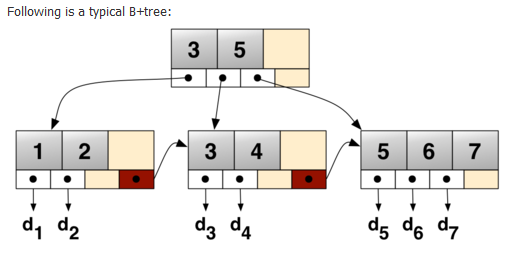Each node of a B+ tree is a page, a block of data. A page is the transfer unit to disk.

We are already aware that a table spans on many blocks of data. We can picture this by having the tree analogous to the table, the nodes analogous to the blocks of data and we have to keep in mind that each block of data contains multiple rows.

For now we’ve talked about two things stored on a disk : the index and the data. The index (in blue below) points at the data (in green below). We clearly notice now that creating an index takes extra space on the disk.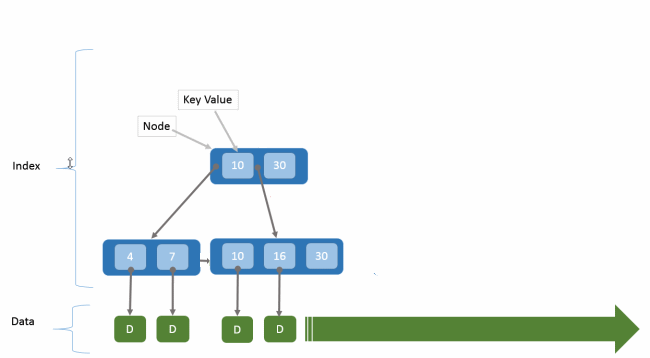Let’s analyze the leaves of a B+ tree. We notice that they are structured as a linked list with 2 pointers:

• one pointer towards the next node
• one pointer towards the data.

Having this tree structure (and not only a sequential linked list structure) helps for insertion and deletion complexities: they have a logarithmic running time.

Say we have a B+ tree with a height h = 2. In this case, 3 blocks of data will be accessed:

• the root
• the leaf holding the pointers
• the data page corresponding to the rows (referenced by the pointers from the leaf)

How do we recognize B+ tree?

Let’s say d is the number of references that a node has to its children.

In order to be a valid B+ tree, it has to respect the following invariants:

• every leaf is at the same distance from the root
• if a node has d pointers, the node has to contain d-1 keys
• every root has at least 2 children
• every non-leaf AND non-root has at least d/2 children
• every leaf contains at least floor d/2 keys
• every key of the column appears in a leaf

Let’s see how does inserting nodes works in a B+ tree. Say we have a node X. The main algorithm is the following :

Step 1: If node X has empty space, insert (key, ref) into the node.

Step 2: If node X already full:

• 2A) split X into 2 nodes : X1 and X2
• 2B) distribute keys evenly between 2 nodes
• 2C) If node X is a leaf : take minimum value of 2nd node X2 and insert in the parent node by repeating the algorithm starting from point 1)
• 2D) if node X is a non-leaf : take minimum value of 2nd node X2, exclude it from the split up and insert it in the parent node by repeating the algorithm starting from point 1)

Let’s go through a few examples. Assume we have 4 rows per page and we’ve inserted the following set of key values : 2, 3, 5, 7, 11, 17, 19, 23, 29, 31.

An empty node with 4 rows per page will look like the following. We notice the 4 empty spaces at the edge for the 4 pointers :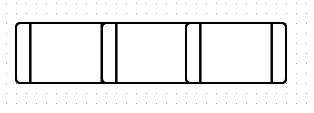1) If we want to insert 2, and then 3, and then 5, we just follow the Step 1 of the algorithm (node has empty space).

2) Now let’s insert 7.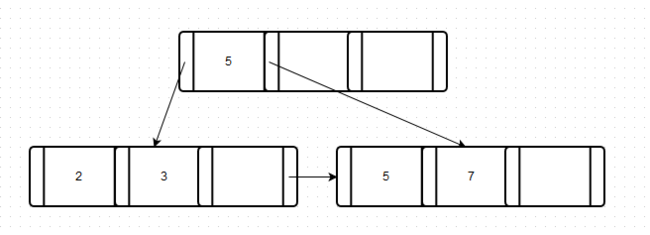What happened?

• Step 2: we notice from part 1 that node X is already full.
• 2A): split node X into 2 nodes : X1 and X2
• 2B): distribute the key evenly between 2 nodes (we have 2 and 3 in node X1 and 5 and 7 in node X2)
• 2C) node X was indeed a leaf : the minimum value of 2nd node X2 is 5. We simply insert it into the parent node by repeating Step 1 from the algorithm (because yes, the parent node has empty space) .
• Step 1 but at the parent’s node: there is space, stop.

3) Let’s insert 11.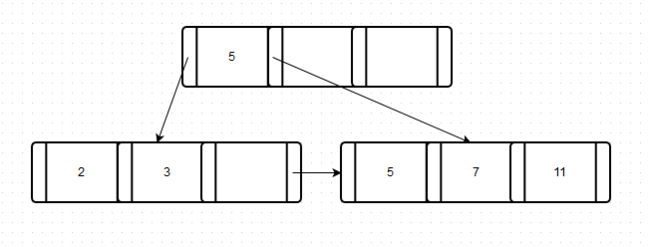What happened?

• Step 1: we notice from part 2 that node X has space.

4) Insert 17.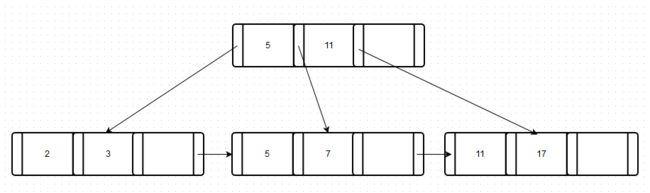What happened?

• we notice from part 3 that there is no more space, hence we continue to step 2
• 2A: we split the node X (containing 5, 7, 11) into 2 nodes
• 2B: we distribute evenly between 2 nodes (we have 5, 7 in X1 and 11, 17 in X2)
• 2C: X was a leaf, hence we take minimum value of 2nd node X2 (11) and insert it into the parent
• Step 1 but at the parent’s node: there is space, stop.

5) Insert 19.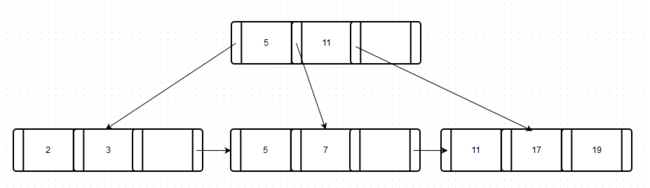What happened?

• Step 1: there was space, we insert and then we stop.

6) Insert 23.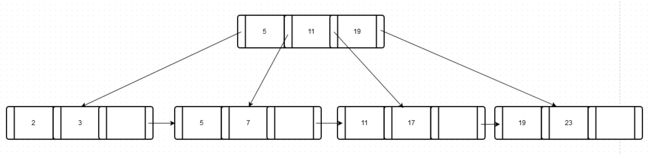What happened?

• Step 1: there is no more space, continue.
• Step 2A: we split the node X (containing 11, 17, 19) into 2 nodes
• Step 2B: we distribute evenly between 2 nodes
• Step 2C: X was a leaf, we take the minimum of 2nd node (19) and we insert it into the parent
• Step 1 but at the parent’s node: there is space, stop.

7) Insert 29.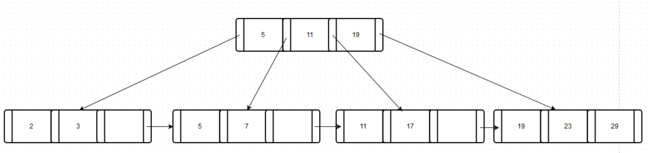What happened?

• Step 1: there was space, we insert and then we stop.

8) Insert 31: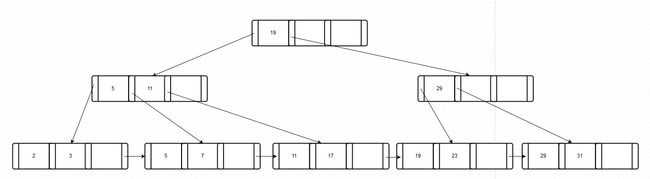What happened?

• step 1 there is no space, continue
• Step 2A: we split the node X (containing 19, 23, 29) into 2 nodes
• Step 2B: we distribute evenly between 2 nodes
• Step 2C: X was a leaf, we take the minimum of 2nd node (29) and we insert it into the parent
• Step 1 but at the parent’s node: there is no space, continue.
• Step 2A: we split the new node X (containing 5, 11, 19) into 2 nodes
• Step 2B: we distribute evenly between 2 nodes
• Step 2D: since the new node X was not a leaf, we exclude the minimum value of the 2nd node (19) and we insert it into the new parent
• Step 1 but at the parent’s node: there is space, stop.

This simulator is pretty neat for testing your own implementations of B+ trees (many thanks to Joy & Graham from New Zealand).

Now why are we using B+ trees?

We notice that, unlike traversing a linked list, accessing any part of the tree requires visiting only a few nodes. Also, increasing the number of child nodes is decreasing the depth of the tree, hence decreasing the number of “hops” (time consuming disk reads) required.